Courses

# Basic Concepts - MCQ Test

## 20 Questions MCQ Test GATE ECE (Electronics) 2022 Mock Test Series | Basic Concepts - MCQ Test

Description
This mock test of Basic Concepts - MCQ Test for Electrical Engineering (EE) helps you for every Electrical Engineering (EE) entrance exam. This contains 20 Multiple Choice Questions for Electrical Engineering (EE) Basic Concepts - MCQ Test (mcq) to study with solutions a complete question bank. The solved questions answers in this Basic Concepts - MCQ Test quiz give you a good mix of easy questions and tough questions. Electrical Engineering (EE) students definitely take this Basic Concepts - MCQ Test exercise for a better result in the exam. You can find other Basic Concepts - MCQ Test extra questions, long questions & short questions for Electrical Engineering (EE) on EduRev as well by searching above.
QUESTION: 1

### A solid copper sphere, 10 cm in diameter is deprived of 1020 electrons by a charging scheme. The charge on the sphere is

Solution:

• n = 1020
• Q = ne
= e * 1020
= -16.02 C
Charge on sphere will be positive as this electric charge is removed.

QUESTION: 2

Solution:

ΔQ = i x Δ t
= 15000 x 100μ
= 1.5 C

QUESTION: 3

### If 120 C of charge passes through an electric conductor in 60 sec, the current in the conductor is

Solution: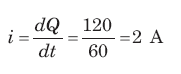QUESTION: 4

The energy required to move 120 coulomb through 3 V is

Solution:

• W = QV
W = 120 * 3
W = 360 J

QUESTION: 5

i = ?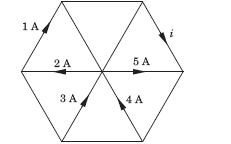Solution: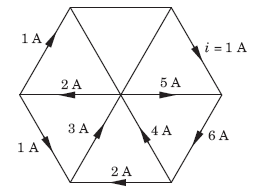Based on kirchoffs current law, we know that incoming currents at a node is equals to outgoing currents.
So, based on this 4 and 3 will get canceled with 5 and 2.
So, finally i = 1.

QUESTION: 6

In the circuit given, a charge of 600 C is delivered to the 100 V source in a 1 minute. The value of v1 must be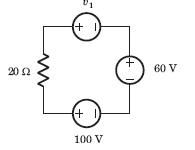Solution:
• In order for 600 C charge to be delivered to the 100 V source, the current must be anticlockwise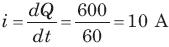Applying KVL we get:
v1 + 60 - 100 = 10 x 20
or, v1 = 240 V

QUESTION: 7

In the circuit of the fig the value of the voltage source E is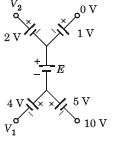Solution:

Going from 10 V to 0 V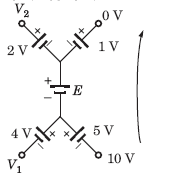10 + 5 + E + 1 = 0

E = -16V

Minus sign indicates that the polarity of battery should be reversed.

QUESTION: 8

Consider the circuit graph shown in fig. Each branch of circuit graph represent a circuit element. The value of voltage v1 is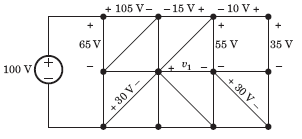Solution:

100 = 65 + v2

⇒ v2 = 35 V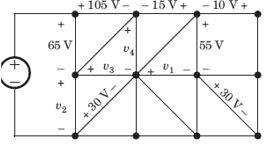v3 - 30 = v2

⇒ v3 = 65 V

105 + v4 - v- 65 = 0

⇒ v4 = 25 V

v4 + 15 - 55 + v1 = 0

⇒ v1 = 15 V

QUESTION: 9

What quantity of charge must be delivered by a battery with a Potential Difference of 110 V to do 660J of Work?

Solution:

► V = W / Q
► Q = W / V
= 660 / 110
= 6C

QUESTION: 10

R1 = ?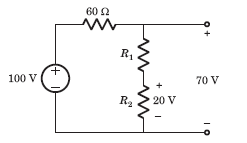Solution:

Voltage across 60 Ω resistor = 30 V
Current = 30 / 60 = 0.5 A
Voltage across R1 is = (70 - 20) V
= 50 V
R1 = 50 / 0.5 = 100 Ω

QUESTION: 11

Twelve 6 Ω resistor are used as edge to form a cube.The resistance between two diagonally opposite corner of the cube is

Solution:

The current i will be distributed in the cube branches symmetrically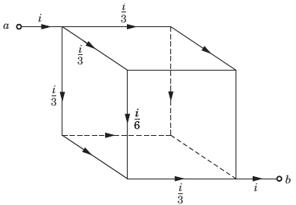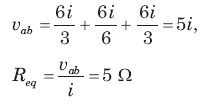QUESTION: 12

v1 = ?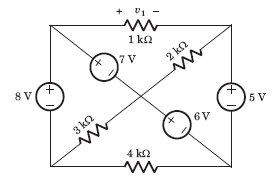Solution:

If we go from +ve side of 1 kΩ through 7 V, 6 V and 5 V
We get,
v1 = 7 + 6 - 5
= 8 V

QUESTION: 13

The voltage vo in fig is always equal to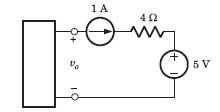Solution:

It is not possible to determine the voltage across 1 A source.

QUESTION: 14

Req =  ?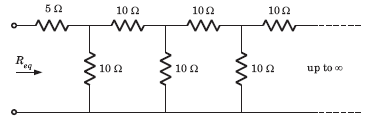Solution:
• We infer from the given diagram that the same pattern is followed after every 10 ohm resistor. The infinite pattern in parallel as a whole is considered as to be Rx.

Thus, Rx = R + (R || Rx)
Solving for Rx, we get Rx = 1.62 R, where R = 10 ohm.
So, we have Rx = (1.62 ohm)*(10 ohm)
This gives Rx = 16.2 ohm
∴ Req = 5 + (10 || 16.2)
⇒ 5 + [(10 * 16.2) / (10 + 16.2)]
⇒  Req = 5 + (162 / 26.2)
which gives Req = 11.18 ohm.

QUESTION: 15

The quantity of a charge that will be transferred by a current flow of 10 A over 1 hour period is_________ ?

Solution:

We know that,
I=Q / t or Q = I x t = 10 x 1 hours
{since unit of current is Cs-1, therefore time should be in seconds}
∴ Q = 10 x 60 x 60
= 36000 C
= 3.6 x 10C

QUESTION: 16

A capacitor is charged by a constant current of 2 mA and results in a voltage increase of 12 V in a 10 sec interval. The value of capacitance is

Solution: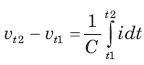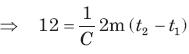12 * C  = 2 m x 10
C = 1.67 mF
Hence, Capacitance is equal to 1.67 mF.

QUESTION: 17

The energy required to charge a 10 μF capacitor to 100 V is

Solution: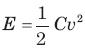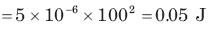QUESTION: 18

The current in a 100 μF capacitor is shown in fig. If capacitor is initially uncharged, then the waveform for the voltage across it is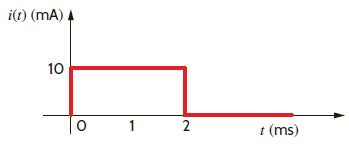Solution: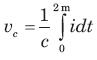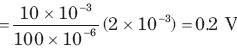This 0.2 V increases linearly from 0 to 0.2 V. Then current is zero. So capacitor hold this voltage.

QUESTION: 19

The voltage across a 100 μF capacitor is shown in fig. The waveform for the current in the capacitor is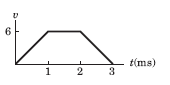Solution: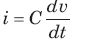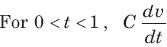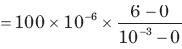= 600 mA
For 1 ms < t < 2 ms,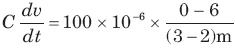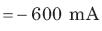QUESTION: 20

The waveform for the current in a 200 μF capacitor is shown in fig. The waveform for the capacitor voltage is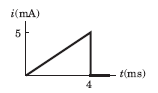Solution: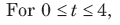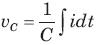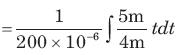= 3125t2
At t = 4 ms, vc = 0.05 V
It will be parabolic path at t = 0
t-axis will be tangent.